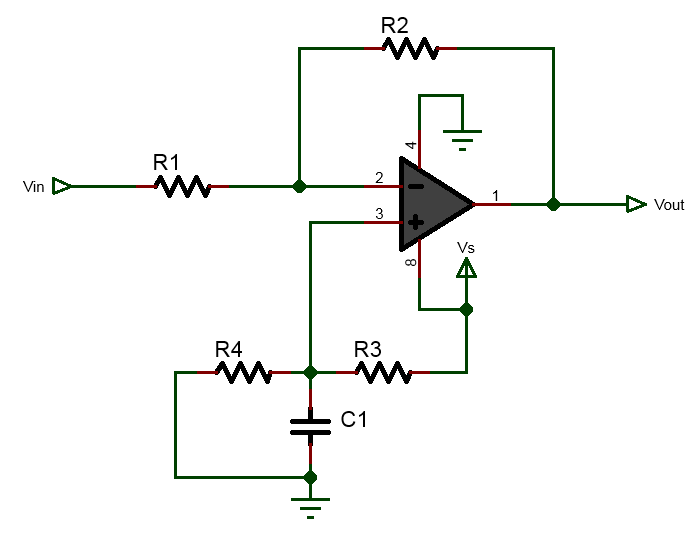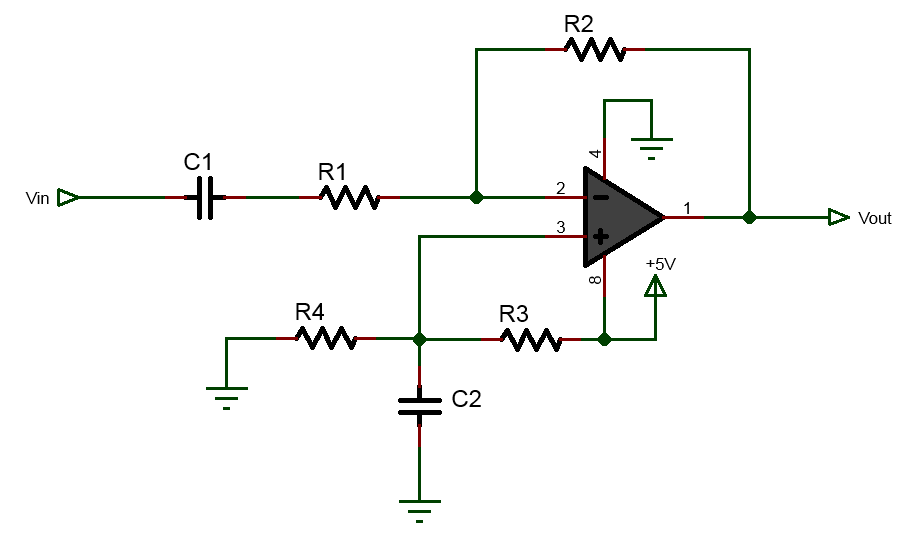# dc coupled inverting op-amp biased with VDB

Inputs:
V

V

Results:## DC coupled Inverting Op-Amp biased with VDB

This calculators helps you to bias an dc coupled inverting op-amp using single supply voltage.

### Equations Used:

$$A_v=-\frac{R_2}{R_1}$$
$$V_b = \frac{R_4}{R_3+R_4}V_s$$

# ac coupled inverting op-amp biased with voltage divider biasing method

Inputs:
V

V

Results:## ac coupled Inverting Op-Amp biased with voltage divider biasing method

This calculator can be used to bias ac coupled inverting op-amp using the split resistor biasing methods.

### Equations Used:

$$A_v=-\frac{R_2}{R_1}$$
$$V_b = \frac{R_4}{R_3+R_4}V_s$$

# ac coupled Inverting Op-Amp biased with voltage follower

Inputs:
V

V

Results:## ac coupled Inverting Op-Amp biased with voltage follower biasing method

The above calculator can be used to bias ac coupled inverting op-amp using voltage follower biasing method.

### Equations Used:

$$A_v=-\frac{R_2}{R_1}$$
$$V_b = \frac{R_4}{R_3+R_4}V_s$$